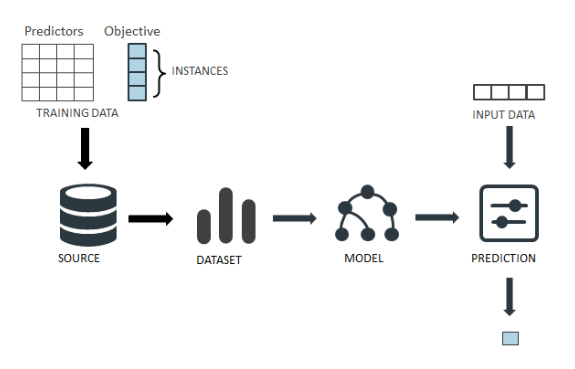Over a million developers have joined DZone.{{announcement.body}}
{{announcement.title}}

DZone's Guide to

# Foundations for Machine Learning and Data Science for Developers

Do you want to learn the fundamentals of data science and machine learning? Check out this brief overview and tutorial.

· Big Data Zone ·
Free Resource

Comment (1)

Save
{{ articles.views | formatCount}} Views

The Architect’s Guide to Big Data Application Performance. Get the Guide.

This tutorial introduces machine learning and data science concepts for developers. On the web, we already have many excellent resources for learning data science, however, the sheer amount of material can, in itself, be daunting. This is based on my insights from the Enterprise AI course and also the Data Science for IoT course which I teach at Oxford University.

This tutorial draws on the content on the web and adds three new elements:

1. We explain concepts simply but in context. Many tutorials explain one specific aspect but do not show how it fits into the wider picture.
2. We approach maths and stats from the perspective/lens of linear regression. This approach has an advantage because linear regression is familiar to many from their high school days.
3. Less is more – we discuss a small number of examples. You can always find more examples of an algorithm type on the web. Future versions of this tutorial will expand on maths and science in context of programming.

I also address a broader question: Which maths and stats techniques do you need for data science?

A knowledge of algorithms (maths and stats) is the main differentiator between traditional programming and analytics-based programming.

Having said that, it helps to start with programming and approach the maths (initially) through APIs and libraries. I find that this technique works better because more people are familiar with programming than with maths.

Here is a list of maths and stats techniques useful for data scientists:

• Linear Algebra (e.g. vector algebra, matrices, transformations, eigenvalues)
• Probability Theory
• Optimization techniques (eg. gradient descent)
• Descriptive Statistics (eg. means, modes, standard deviations, variances, distributions)

Techniques used in data science such as data transformations, exploratory data analysis, feature engineering, ensemble strategies, and visualization (story telling) all involve maths and stats.

## An Overview of Data Science & Machine Learning

Data Science is a multidisciplinary domain involved in solving analytically complex problems using machine learning algorithms. Unlike traditional algorithms, machine learning algorithms are data driven. Thus, the data determines the algorithm’s response. For example, for an application to detect photos, a non-machine learning algorithm would try to first define what a face is. In contrast, a machine learning algorithm would not have such a pre-determined definition but rather it would "learn-by-examples."

Data science includes a formal set of steps. Hilary Mason and Chris Wiggins describe a process called OSEMN which is summarized as:

1. Obtain data
2. Scrub data
3. Explore data:‘Explore’ in this context refers to exploratory data analysis; i.e. no hypothesis that is being tested and no predictions that are being evaluated.
4. Model data (machine learning stage): Here, we apply the predictive models (regression, classification,etc.). A model’s predictive accuracy can be evaluated by how well it performs on unseen data.
5. Interpret results

Broadly, we will also follow this sequence in this tutorial.

## What Is Machine Learning?

As we see above, Machine learning can be seen as a step in the wider data science process. Tom Mitchell says this in his book Machine Learning:

“The field of machine learning is concerned with the question of how to construct computer programs that automatically improve with experience.”

The formal definition of machine learning is as follows:

“A computer program is said to learn from experience E with respect to some class of tasks T and performance measure P, if its performance at tasks in T, as measured by P, improves with experience E.”

From a programmer’s perspective machine learning involves:

1. Training of a model from data.
2. Predict/extrapolate a decision.
3. Measure against a performance measure.

Visually, we can see this process as:Check out the tutorial here.

Learn how taking a DataOps approach will help you speed up processes and increase data quality by providing streamlined analytics pipelines via automation and testing. Learn More.

Topics:
data science ,machine learning ,statistics ,big data ,tutorial

Comment (1)

Save
{{ articles.views | formatCount}} Views

Opinions expressed by DZone contributors are their own.

# {{ parent.title || parent.header.title}}

{{ parent.tldr }}

{{ parent.urlSource.name }}